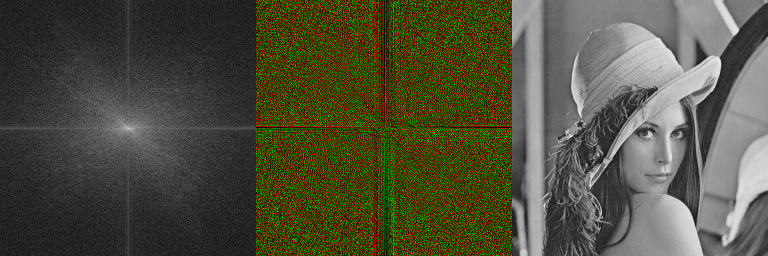# Example Discrete Fourier TransformLeft: Magnitude of DCF. Middle: Phase of DCF. Right: Input image

Discrete Fourier Transform (DCF) is widely in image processing. The fast fourier transform (FFT) allows the DCF to be used in real time and runs much faster if the width and height are both powers of two. BoofCV provides operators for manipulating the DCF and for visualizating the results, as this example shows.

Example Code:

Concepts:

• Fourier Transform
• Frequency Domain Filtering

# Example Code

```/**
* Example demonstrating how to compute the Discrete Fourier Transform, visualize the transform, and apply
* a filter frequency domain.
*
* @author Peter Abeles
*/
public class ExampleFourierTransform {

/**
* Demonstration of how to apply a box filter in the frequency domain and compares the results
* to a box filter which has been applied in the spatial domain
*/
public static void applyBoxFilter( GrayF32 input ) {

// declare storage
GrayF32 boxImage = new GrayF32(input.width, input.height);
InterleavedF32 boxTransform = new InterleavedF32(input.width,input.height,2);
InterleavedF32 transform = new InterleavedF32(input.width,input.height,2);
GrayF32 blurredImage = new GrayF32(input.width, input.height);
GrayF32 spatialBlur = new GrayF32(input.width, input.height);

DiscreteFourierTransform<GrayF32,InterleavedF32> dft =
DiscreteFourierTransformOps.createTransformF32();

// Make the image scaled from 0 to 1 to reduce overflow issues
PixelMath.divide(input,255.0f,input);

// compute the Fourier Transform
dft.forward(input,transform);

// create the box filter which is centered around the pixel.  Note that the filter gets wrapped around
// the image edges
for( int y = 0; y < 15; y++ ) {
int yy = y-7 < 0 ? boxImage.height+(y-7) : y - 7;
for( int x = 0; x < 15; x++ ) {
int xx = x-7 < 0 ? boxImage.width+(x-7) : x - 7;
// Set the value such that it doesn't change the image intensity
boxImage.set(xx,yy,1.0f/(15*15));
}
}

// compute the DFT for the box filter
dft.forward(boxImage,boxTransform);

// Visualize the Fourier Transform for the input image and the box filter
displayTransform(transform,"Input Image");
displayTransform(boxTransform,"Box Filter");

// apply the filter. convolution in spacial domain is the same as multiplication in the frequency domain
DiscreteFourierTransformOps.multiplyComplex(transform,boxTransform,transform);

// convert the image back and display the results
dft.inverse(transform,blurredImage);
// undo change of scale
PixelMath.multiply(blurredImage,255.0f,blurredImage);
PixelMath.multiply(input,255.0f,input);

// For sake of comparison, let's compute the box blur filter in the spatial domain
// NOTE: The image border will be different since the frequency domain wraps around and this implementation
// of the spacial domain adapts the kernel size
BlurImageOps.mean(input,spatialBlur,7,null,null);

// Convert to BufferedImage for output
BufferedImage originOut = ConvertBufferedImage.convertTo(input, null);
BufferedImage spacialOut = ConvertBufferedImage.convertTo(spatialBlur, null);
BufferedImage blurredOut = ConvertBufferedImage.convertTo(blurredImage, null);

ListDisplayPanel listPanel = new ListDisplayPanel();

ShowImages.showWindow(listPanel,"Box Blur in Spacial and Frequency Domain of Input Image");
}

/**
* Display the fourier transform's magnitude and phase.
*/
public static void displayTransform( InterleavedF32 transform , String name ) {

// declare storage
GrayF32 magnitude = new GrayF32(transform.width,transform.height);
GrayF32 phase = new GrayF32(transform.width,transform.height);

// Make a copy so that you don't modify the input
transform = transform.clone();

// shift the zero-frequency into the image center, as is standard in image processing
DiscreteFourierTransformOps.shiftZeroFrequency(transform,true);

// Compute the transform's magnitude and phase
DiscreteFourierTransformOps.magnitude(transform,magnitude);
DiscreteFourierTransformOps.phase(transform, phase);

// Convert it to a log scale for visibility
PixelMath.log(magnitude,magnitude);

// Display the results
BufferedImage visualMag = VisualizeImageData.grayMagnitude(magnitude, null, -1);
BufferedImage visualPhase = VisualizeImageData.colorizeSign(phase, null, Math.PI);

ImageGridPanel dual = new ImageGridPanel(1,2,visualMag,visualPhase);
ShowImages.showWindow(dual,"Magnitude and Phase of "+name);
}

public static void main( String args[] ) {

GrayF32 input = UtilImageIO.loadImage(UtilIO.pathExample("standard/kodim17.jpg"), GrayF32.class);

applyBoxFilter(input.clone());
}
}
```